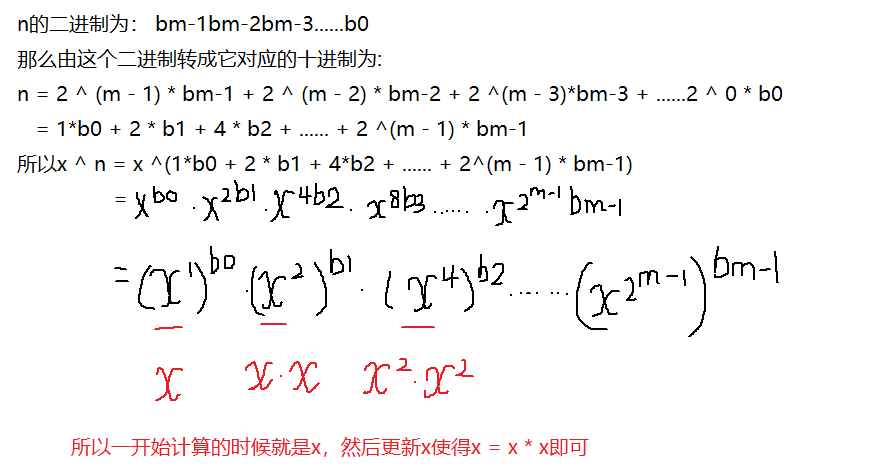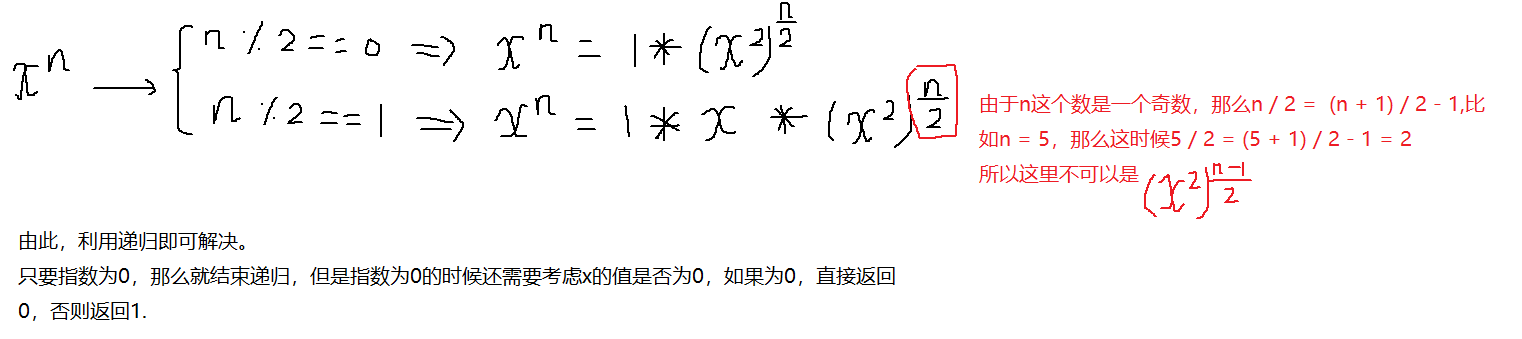﻿• 价格透明
• 信息保密
• 进度掌控
• 售后无忧# LeetCode 数值的整数次方

-100.0 < x < 100.0
-231 <= n <= 231-1
-104 <= xn <= 104``````class Solution {

public double myPow(double x, int n) {
if(n == 0){
//如果n为0，那么需要判断x是否为0，如果为0，返回0，否则返回1
if(x == 0)
return 0;
else return 1;
}else{
//如果n不为0，那么就计算
/*
这一步十分重要，如果没有这一步，那么n的值是-2147483648(即int
的最小值)，由于是小于0，那么需要将n化成正数，但是一旦化成正
数，就会导致n的值变成无穷小，最后在下面循环的时候就会陷入死循
环，从而超时。所以为了避免这种情况，需要将n的值赋值给一个long
类型的变量，这样即使n的值是int的最小值，也不会发生越界
*/
long b = n;
if(n < 0){
b = -b;
// n = -n;
// System.out.println(n);
x = 1/x;
}
int res;
double result = 1;
while(b != 0){
//由于b已经是正数，那么无论是右移还是左移，都是补0，所以while循环条件就是判断b是否为0
res = (int)b & 1;
result = result * Math.pow(x,res);
x = x * x;//更新x
b = b >> 1;
}
return result;
}
}
}
````````````class Solution {
/*
public double myPow(double x, int n) {
if(n == 0){
//如果n为0，那么需要判断x是否为0，如果为0，返回0，否则返回1
if(x == 0)
return 0;
else return 1;
}else{
//如果n不为0，那么就计算

这一步十分重要，如果没有这一步，那么n的值是-2147483648(即int的最小值)，由于是
是小于0，那么需要将n化成正数，但是一旦化成正数，就会导致n的值变成无穷小，最后在下面循环
的时候就会陷入死循环，从而超时。所以为了避免这种情况，需要将n的值赋值给一个long类型的
变量，这样即使n的值是int的最小值，也不会发生越界

long b = n;
if(n < 0){
b = -b;
// n = -n;
// System.out.println(n);
x = 1/x;
}
int res;
double result = 1;
int count = 1;
while(b != 0){
//由于b已经是正数，那么无论是右移还是左移，都是补0，所以while循环条件就是判断b是否为0
res = (int)b & 1;
result = result * Math.pow(x,res);
x = x * x;
b = b >> 1; //由于b已经是正数，那么无论是右移还是左移，都是补0，所以while循环条件就是判断b是否为0
}
return result;
}
}

*/
public double myPow(double x, int n) {
if(n == 0){
//如果n为0，那么需要判断x是否为0，如果为0，返回0，否则返回1
if(x == 0)
return 0;
else return 1;
}else{
/*
如果n不为0，那么即利用二分求幂进行求解
即将n = n / 2 + n / 2，从而x^n = x ^(n / 2 * 2) = (x ^ 2) ^
(n / 2)但是需要考虑的是当前的n是否能够化成两个相等的数相加（即
需要判断奇偶性）如果n是一个偶数，那么最后的结果就是(x ^ 2) ^
(n / 2),此时需要递归，返回的结果是1 * myPow(x ^ 2,n / 2)
否则，如果n是一个奇数，那么就需要取出一个x，然后对n - 1个x进行
平方,即返回的结果是1 * x * myPow(x ^ 2,n / 2)
注意不是(n - 1) / 2
*/
long b = n;
if(b < 0){
b = -b;
x = 1 / x;
}
if(b % 2 == 0){
return myPow(x * x,(int)(b / 2));
}else{
return x * myPow(x * x,(int)( b / 2)); //注意，这里需要是(int)(b / 2),否则就会发生报错，其次不应该是(b - 1) / 2
}

}
}
}
``````### 低价透明### 金牌服务### 信息保密